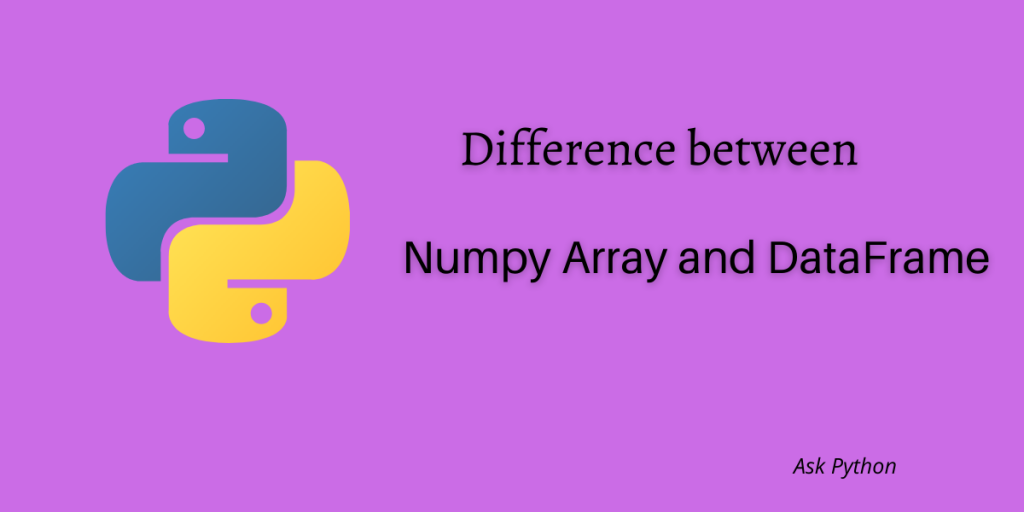# Difference Between Pandas Dataframe and Numpy ArraysWe often get confused between data structures in Python as they may seem kind of similar. DataFrame and arrays in Python are two very important data structures and are useful in data analysis. In this article, we are going to learn about the differences between Pandas DataFrame and Numpy Array in Python.

Let’s start by understanding Numpy arrays.

Also read: Converting Pandas DataFrame to Numpy Array [Step-By-Step]

## What Is a Numpy Array?

A NumPy array is a type of multi-dimensional data structure in Python which can store objects of similar data types. The elements of the array are indexed by non-negative or positive integers. Arrays are mutable which means arrays can be changed after it is being formed. Arrays are a lot useful for performing mathematical operations on vectors. They provide a lot of useful methods for performing vector operations.

Let’s see how we can create an array.

We will be using the Numpy library in Python.

```import numpy as np
arr = np.array([1, 2, 3, 4, 5])
print(arr)

```

Output:

```[1, 2, 3,4, 5]
```

Now let’s see what Pandas DataFrame is.

## What Is a Dataframe?

DataFrame is a two-dimensional, tabular, mutable data structure in Python that can store tabular data containing objects of different data types. A DataFrame has labeled axes in the form of rows and columns. DataFrames are useful tools in data pre-processing as it provides useful methods for data handling.DataFrames are also very useful for creating pivot tables and plotting with Matplotlib.

Let’s see how we can create a DataFrame in Pandas.

```import pandas as pd
# Creating a dictionary
data = {'Name':["Tommy","Linda","Justin","Brendon"], 'Age':[31,24,16,22]}
df=pd.DataFrame(data)
print(df)
```

Output:

```      Name    Age
0    Tommy   31
1    Linda   24
2   Justin   16
3  Brendon   22
```

## Comparison between DataFrame and Array

The major differences between DataFrame and Array are listed below:

1. Numpy arrays can be multi-dimensional whereas DataFrame can only be two-dimensional.
2. Arrays contain similar types of objects or elements whereas DataFrame can have objects or multiple or similar data types.
3. Both array and DataFrames are mutable.
4. Elements in an array can be accessed using only integer positions whereas elements in a DataFrame can be accessed using both integer and index positions.
5. DataFrames are mostly in the form of SQL tables and are associated with tabular data whereas arrays are associated with numerical data and computation.
6. DataFrames can deal with dynamic data and mixed data types whereas arrays do not have the flexibility to handle such data.

## Conclusion

In this post, you learned the differences between Pandas DataFrame and Numpy Array. Numpy arrays are specifically used when complex scientific computation has to be performed whereas DataFrames are used mostly in data pre-processing. Although both of these data structures play a very important role in data analysis.